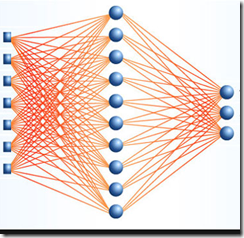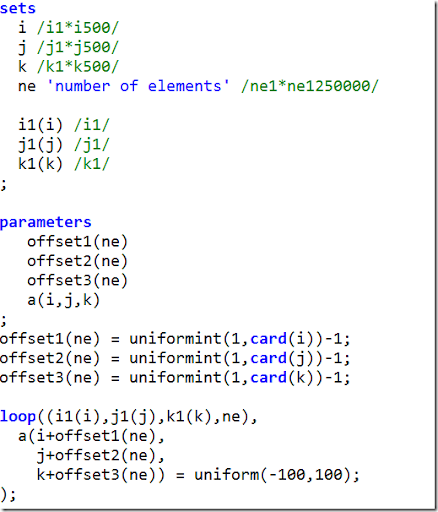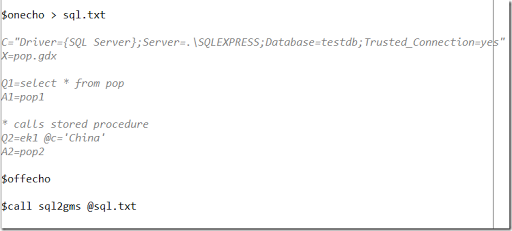## Thursday, June 27, 2013

### Calling R from GAMS to produce high quality graphs

Here is a small demo script that shows how we can call R from GAMS to produce graphs using ggplot.

 * name of the R script to execute \$set rscript  my_script.R * include file with data \$include popage.inc execute_unload 'popage.gdx'; execute 'gdxdump popage.gdx format=csv symb=popage output=popage.csv'; execute 'head popage.csv'; execute '"C:\Program Files\R\R-2.15.0\bin\R.exe" --vanilla < %rscript%'; execute 'shellexecute areaplot.svg'; execute 'shellexecute percentplot.svg'; \$onecho > %rscript% popage = read.csv("popage.csv") popage\$AgeGroup = factor(popage\$AgeGroup, levels = c('<5','5-14','15-24','25-34','35-44','45-54','55-64','>64')) summary(popage) library(ggplot2) svg("areaplot.svg") ggplot(popage, aes(x=Year, y=Val, fill=AgeGroup))  + geom_area() + ylab("Population (Thousands)") dev.off() library(plyr) popage_percent = ddply(popage, "Year", transform, Percent = Val / sum(Val) * 100) svg("percentplot.svg") ggplot(popage_percent, aes(x=Year, y=Percent, fill=AgeGroup))  +     geom_area(colour='black', size=.2, alpha=.4) +     scale_fill_brewer(palette='Blues', breaks=rev(levels(popage\$AgeGroup))) dev.off() \$offecho

Results look like:

We reproduced these graphs from from:## Monday, June 17, 2013

### Neural Networks in GAMSI was running some neural networks with a back propagation algorithm using a rather simple optimization tool (gradient descent). This actually ran pretty decent.  Now lets if we can formulate something like this in GAMS. I tried something like:

I know the formulation is not great but may be better high-performance sparse solvers would help me out here and still do a decent job. Unfortunately, the result was dramatically poor. Most solvers ran out of memory, and MINOS was still running after 3 hours.

The basic problem is that we really only want THETA2 and THETA3 as variables and all the rest just as temporary intermediate values. That makes the problem very much smaller (the thetas are not depending on i which is the observation number of the training set). With AMPL we probably could have used defined variables to make this work but GAMS does not offer this.

## Thursday, June 13, 2013

### Creating sparse data

To test certain modeling constructs it may be useful to generate some random data. In GAMS this is usually done through something like:

a(i,j,k) = uniform(-100,100);

When we want to have a sparse data set we can use the following:

a(i,j,k) = uniform(-100,100)\$(uniform(0,1)<0.1);

i.e. with probability 0.1 generate an entry in A with a random value between -100 and 100.

This can also be written as:

a(i,j,k)\$(uniform(0,1)<0.1) = uniform(-100,100);

For very large, very sparse structures it may be even better to generate a random sparsity pattern first. E.g. using something like:## Thursday, June 6, 2013

### RAS Algorithm Refinement

In the post http://yetanothermathprogrammingconsultant.blogspot.com/2013/06/simple-data-set-to-show-equivalence-of.html a simple RAS algorithm was shown. A slightly better way is to use a convergence criterion.

I got some feedback that users were not really familiar with how to write this in GAMS. So I thought this would be a good thing to share.

 \$ontext    Example from    Using PROC IML to do Matrix Balancing    Carol Alderman, University of Kansas    Institute for Public Policy and Business Research    MidWest SAS Users Group MWSUG 1992    In this version we stop on convergence \$offtext sets    p 'products' /pA*pI/    s 'salesmen' /s1*s10/ ; table A0(*,*) 'estimated matrix, known totals'             s1   s2   s3   s4   s5   s6   s7   s8   s9  s10   rowTotal       pA   230  375  375  100    0  685  215    0   50    0       2029       pB   330  405  419  175   90  504  515    0  240  105       2798       pC   268  225  242    0   30  790  301   44  100    0       1998       pD   595  380  638  275   30  685  605   88  100  160       3566       pE   340  360  440  200   30  755  475   44  150    0       2794       pF   132  190  200    0    0  432  130    0    0    0       1071       pG   309  330  350  125    0  612  474    0   50   50       2305       pH   365  400  330  150   50  575  600   44  150  110       2747       pI   210  250  308  125    0  720  256    0  100   50       2015 colTotal  2772 2910 3300 1150  240 5760 3526  220  950  495 ; A0(p,'sum') = sum(s,A0(p,s)); A0('sum',s) = sum(p,A0(p,s)); A0(p,'diff') = A0(p,'sum') - A0(p,'rowTotal'); A0('diff',s) = A0('sum',s) - A0('colTotal',s); option A0:0; display A0; alias (p,i); alias (s,j); parameters    A(*,*)  'new RASsed matrix'    u(i)    'row total'    v(j)    'column total'    rho(i)   'used inside RAS algorithm'    sigma(j) 'used inside RAS algorithm' ; A(i,j) = A0(i,j); u(i) = A0(i,'rowTotal'); v(j) = A0('colTotal',j); abort\$(smin((i,j),A(i,j))<0) "Entries A(i,j) should be nonnegative",A; abort\$(smin(i,u(i))<0) "Entries u(i) should be nonnegative",u; abort\$(smin(j,v(j))<0) "Entries v(j) should be nonnegative",v; set iter 'max iterations of RAS algorithm' /iter1*iter100/; scalars    continue /1/    tol /1e-7/    diff    count ; loop(iter\$continue, * * step 1 row scaling *    rho(i) = u(i)/sum(j,A(i,j));    A(i,j) = rho(i)*A(i,j); * * step 2 column scaling *    sigma(j) = v(j)/sum(i,A(i,j));    A(i,j) =  A(i,j)*sigma(j); * * check for convergence *    diff = max(smax(i,abs(rho(i)-1)),smax(j,abs(sigma(j)-1)));    continue\$(diff

I often use a large set to loop over: “loop(iter,”. This will make sure we always terminate (no infinite loop). The \$ condition on the loop can be used to terminate the loop when convergence is observed: “loop(iter\$continue,”. Finally we easily can check whether the loop terminated with or without convergence and give an appropriate message.

### SQL2GMS and Stored Procedures

Yes, that works. See:The stored procedure should return a result set which SQL2GMS will then store in the GDX file.

## Monday, June 3, 2013

### GRAS (Generalized RAS) Example

 \$ontext     Generalized RAS method     THEO JUNIUS & JAN OOSTERHAVEN     The Solution of Updating or Regionalizing a Matrix     with both Positive and Negative Entries     Economic Systems Research, Vol. 15, No. 1, 2003     Lenzen, M., Wood, R. and Gallego, B. (2007)     Some comments on the GRAS method,     Economic Systems Research, 19, pp. 461–465 \$offtext table IO_0(*,*) 'Initial, old input–output table'               Goods  Services  Consumption  NetExports TotalOutput Goods           7      3            5         -3           12 Services        2      9            8          1           20 NetTaxes       -2      0            2          1            1 TotalUse        7     12           15         -1           33 ValueAdded      5      8            0          0           13 TotalInput     12     20           15         -1 ; table IO_1(*,*)  'Row and column totals of the new, to be updated, input–output table'               Goods  Services  Consumption  NetExports TotalOutput Goods                                                      15 Services                                                   26 NetTaxes                                                   -1 TotalUse        9     16           17         -2           40 ValueAdded      6     10            0          0           16 TotalInput     15     26           17         -2 ; sets   i /Goods,Services,NetTaxes/   j /Goods,Services,Consumption,NetExports/ ; parameter    a(i,j)  'old matrix'    u(i)    'new row sum'    v(j)    'new column sum'    e       '1 or exp(1) depending on paper' ; a(i,j) = IO_0(i,j); u(i) = IO_1(i,'TotalOutput'); v(j) = IO_1('TotalUse',j); * Junius/Oosterhaven: * e = 1; * Lenzen,e.a.: e = exp(1); display a,u,v; variables    z(i,j)   'note: z(i,j)=x(i,j)/a(i,j)'    obj ; equations    objective    rowsum(i)    colsum(j) ; objective.. obj =e= sum((i,j),abs(a(i,j))*z(i,j)*log(z(i,j)/e)); rowsum(i).. sum(j,a(i,j)*z(i,j)) =e= u(i); colsum(j).. sum(i,a(i,j)*z(i,j)) =e= v(j); z.L(i,j) = 1; z.lo(i,j) = 0.0001; model m /all/; solve m using nlp minimizing obj; IO_1(i,j) = z.l(i,j)*a(i,j); display IO_0,IO_1;

Notes:

• We can declare z(i,j)=x(i,j)/a(i,j) as a positive variable.
• This makes things sign preserving.
• z(i,j)’s will not be generated if a(i,j)=0. Some papers mention we should set z(i,j)=1 if a(i,j)=0 but here we just omit all these entries.
• In the results below GAMS is truncating labels while there is in fact enough room to print them completely (or: counting is difficult!). I even reported this little fact to the GAMS people.

Results:

 ----     89 PARAMETER IO_0  Initial, old input–output table                  Goods    Services  Consumpti~  NetExports  TotalOutp~ Goods            7.000       3.000       5.000      -3.000      12.000 Services         2.000       9.000       8.000       1.000      20.000 NetTaxes        -2.000                   2.000       1.000       1.000 TotalUse         7.000      12.000      15.000      -1.000      33.000 ValueAdded       5.000       8.000                              13.000 TotalInput      12.000      20.000      15.000      -1.000 ----     89 PARAMETER IO_1  Row and column totals of the new, to be updated, input–output table                  Goods    Services  Consumpti~  NetExports  TotalOutp~ Goods            8.976       3.743       5.722      -3.441      15.000 Services         2.799      12.257       9.992       0.952      26.000 NetTaxes        -2.776                   1.286       0.490      -1.000 TotalUse         9.000      16.000      17.000      -2.000      40.000 ValueAdded       6.000      10.000                              16.000 TotalInput      15.000      26.000      17.000      -2.000

## Saturday, June 1, 2013

### Simple Data Set to show equivalence of RAS algorithm and Entropy model

For the matrix balancing problem it is well known that the RAS algorithm and an Entropy model give the same results. Here is a small example with the data taken from http://www.lexjansen.com/mwsug/1992/MWSUG92013.pdf.

In the models below the row and column totals are known while the inner part of the matrix is estimated. The goal is to find a nearby matrix such that the row and column sums are equal to the given row and column totals. For many economic problems it is important we preserve the zeros.

The RAS algorithm implementation can easily be improved by iterating until convergence is observed instead of the fixed number of iterations. One big advantage of using an Entropy model instead of an RAS-like algorithm is that it is easy to add side-constraints. This can often be very important in practical situations.

### RAS Algorithm

 \$ontext    Example from    Using PROC IML to do Matrix Balancing    Carol Alderman, University of Kansas    Institute for Public Policy and Business Research    MidWest SAS Users Group MWSUG 1992 \$offtext sets    p 'products' /pA*pI/    s 'salesmen' /s1*s10/ ; table A0(*,*) 'estimated matrix, known totals'             s1   s2   s3   s4   s5   s6   s7   s8   s9  s10   rowTotal       pA   230  375  375  100    0  685  215    0   50    0       2029       pB   330  405  419  175   90  504  515    0  240  105       2798       pC   268  225  242    0   30  790  301   44  100    0       1998       pD   595  380  638  275   30  685  605   88  100  160       3566       pE   340  360  440  200   30  755  475   44  150    0       2794       pF   132  190  200    0    0  432  130    0    0    0       1071       pG   309  330  350  125    0  612  474    0   50   50       2305       pH   365  400  330  150   50  575  600   44  150  110       2747       pI   210  250  308  125    0  720  256    0  100   50       2015 colTotal  2772 2910 3300 1150  240 5760 3526  220  950  495 ; A0(p,'sum') = sum(s,A0(p,s)); A0('sum',s) = sum(p,A0(p,s)); A0(p,'diff') = A0(p,'sum') - A0(p,'rowTotal'); A0('diff',s) = A0('sum',s) - A0('colTotal',s); option A0:0; display A0; alias (p,i); alias (s,j); parameters    A(*,*)  'new RASsed matrix'    u(i)    'row total'    v(j)    'column total'    rho(i)   'used inside RAS algorithm'    sigma(j) 'used inside RAS algorithm' ; A(i,j) = A0(i,j); u(i) = A0(i,'rowTotal'); v(j) = A0('colTotal',j); abort\$(smin((i,j),A(i,j))<0) "Entries A(i,j) should be nonnegative",A; abort\$(smin(i,u(i))<0) "Entries u(i) should be nonnegative",u; abort\$(smin(j,v(j))<0) "Entries v(j) should be nonnegative",v; set iter 'iterations of RAS algorithm' /iter1*iter10/; loop(iter, * * step 1 row scaling *    rho(i) = u(i)/sum(j,A(i,j));    A(i,j) = rho(i)*A(i,j); * * step 2 column scaling *    sigma(j) = v(j)/sum(i,A(i,j));    A(i,j) =  A(i,j)*sigma(j); ); A(i,'rowTotal') = u(i); A('colTotal',j) = v(j); A(p,'sum') = sum(s,A(p,s)); A('sum',s) = sum(p,A(p,s)); A(p,'diff') = A(p,'sum') - A(p,'rowTotal'); A('diff',s) = A('sum',s) - A('colTotal',s); display "-------------------------------------------------------------------",         "Results",         "-------------------------------------------------------------------",         A;

### Entropy Model

 \$ontext    Example from    Using PROC IML to do Matrix Balancing    Carol Alderman, University of Kansas    Institute for Public Policy and Business Research    MidWest SAS Users Group MWSUG 1992 \$offtext sets    p 'products' /pA*pI/    s 'salesmen' /s1*s10/ ; table A0(*,*) 'estimated matrix, known totals'             s1   s2   s3   s4   s5   s6   s7   s8   s9  s10   rowTotal       pA   230  375  375  100    0  685  215    0   50    0       2029       pB   330  405  419  175   90  504  515    0  240  105       2798       pC   268  225  242    0   30  790  301   44  100    0       1998       pD   595  380  638  275   30  685  605   88  100  160       3566       pE   340  360  440  200   30  755  475   44  150    0       2794       pF   132  190  200    0    0  432  130    0    0    0       1071       pG   309  330  350  125    0  612  474    0   50   50       2305       pH   365  400  330  150   50  575  600   44  150  110       2747       pI   210  250  308  125    0  720  256    0  100   50       2015 colTotal  2772 2910 3300 1150  240 5760 3526  220  950  495 ; A0(p,'sum') = sum(s,A0(p,s)); A0('sum',s) = sum(p,A0(p,s)); A0(p,'diff') = A0(p,'sum') - A0(p,'rowTotal'); A0('diff',s) = A0('sum',s) - A0('colTotal',s); option A0:0; display A0; alias (p,i); alias (s,j); variables A(i,j),z; equations    objective    rowsum(i)    colsum(j) ; objective.. z =e= sum((i,j)\$A0(i,j), A(i,j)*log(A(i,j)/A0(i,j))); rowsum(i).. sum(j\$A0(i,j), A(i,j)) =e= A0(i,'rowTotal'); colsum(j).. sum(i\$A0(i,j), A(i,j)) =e= A0('colTotal',j); A.L(i,j) = A0(i,j); A.lo(i,j)\$A0(i,j) = 0.0001; model m /all/; solve m minimizing z using nlp; parameter A1(*,*); A1(i,j) = A.L(i,j); A1(i,'rowTotal') = A0(i,'rowTotal'); A1('colTotal',j) = A0('colTotal',j); A1(p,'sum') = sum(s,A1(p,s)); A1('sum',s) = sum(p,A1(p,s)); A1(p,'diff') = A1(p,'sum') - A1(p,'rowTotal'); A1('diff',s) = A1('sum',s) - A1('colTotal',s); display "-------------------------------------------------------------------",         "Results",         "-------------------------------------------------------------------",         A1;

### Results

 ----     73 -------------------------------------------------------------------             Results             ------------------------------------------------------------------- ----     73 PARAMETER A1                    s1          s2          s3          s4          s5          s6          s7 PA           229.652     374.869     375.099     100.028                 686.238     212.542 PB           330.587     406.192     420.492     175.626      94.300     506.575     510.790 PC           267.313     224.685     241.809                  31.297     790.595     297.246 PD           595.262     380.610     639.416     275.614      31.391     687.580     599.252 PE           339.659     360.058     440.341     200.158      31.346     756.750     469.809 PF           130.330     187.815     197.821                             427.953     127.080 PG           309.478     330.895     351.165     125.418                 614.984     470.016 PH           360.594     395.631     326.596     148.455      51.665     569.947     586.867 PI           209.123     249.246     307.260     124.701                 719.378     252.398 colTotal    2772.000    2910.000    3300.000    1150.000     240.000    5760.000    3526.000 sum         2772.000    2910.000    3300.000    1150.000     240.000    5760.000    3526.000 diff     -9.0949E-13             -4.5475E-13 -2.2737E-13 -2.8422E-14 -9.0949E-13 -9.0949E-13        +          s8          s9         s10    rowTotal         sum        diff PA                        50.572                2029.000    2029.000 -2.2737E-13 PB                       243.545     109.894    2798.000    2798.000 PC            44.017     101.037                1998.000    1998.000 PD            88.299     101.341     167.233    3566.000    3566.000 -1.3642E-12 PE            44.086     151.793                2794.000    2794.000 PF                                              1071.000    1071.000 PG                        50.727      52.318    2305.000    2305.000 4.54747E-13 PH            43.598     150.111     113.535    2747.000    2747.000 PI                       100.874      52.019    2015.000    2015.000 -4.5475E-13 colTotal     220.000     950.000     495.000 sum          220.000     950.000     495.000 diff     5.68434E-14 1.13687E-13 -5.6843E-14